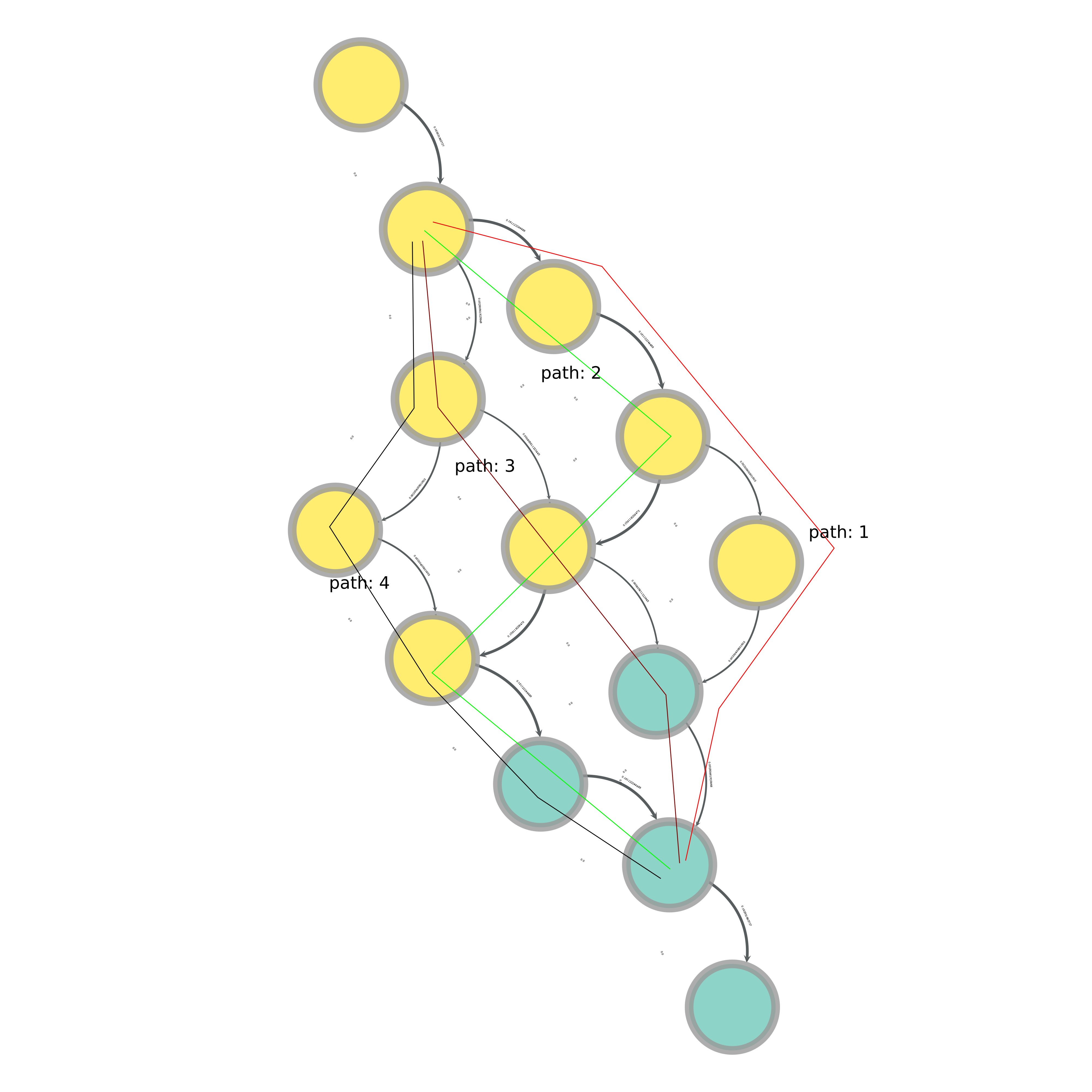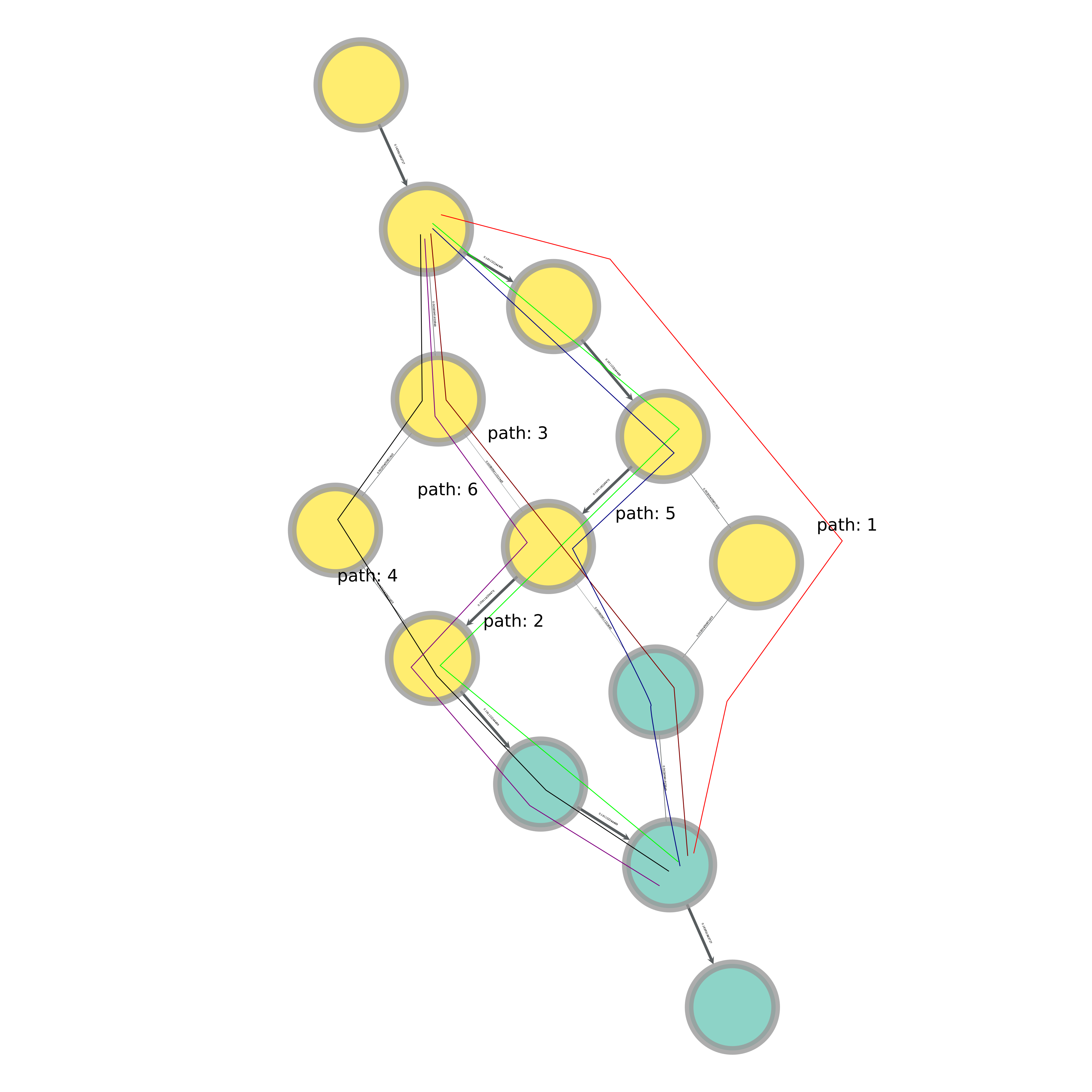# FW: Graphtool: Finding the paths that the maximum flow algorithm produces

Hi Tiago and everyone else on the list

The attached png is one example of a max flow calculation result.
The flow is limited in four places in this graph.
I would like to extract the order of the edges in the four paths I drew.

The shortest path solution you described corresponds to path 3 in the drawing.
all_shortest_paths gives my path 3 and another completely different path before crashing with a memoryError.

Looking forward to hearing from you.
Best,

AlexHi Tiago and everyone else on the list

The attached png is one example of a max flow calculation result.
The flow is limited in four places in this graph.

I'll have to trust you. It is not possible to tell from the image that there
are only four possible paths.

I would like to extract the order of the edges in the four paths I drew.

The shortest path solution you described corresponds to path 3 in the drawing.
all_shortest_paths gives my path 3 and another completely different path before crashing with a memoryError.

I'm not sure why you get a memory error. I can only guess, since you are not
giving me a concrete example of what you are trying to do (i.e. code).

If you are not filtering the graph, or if there are more than 4 possible
paths, you may run out of memory if you try to store them all. Note that
even if there are 4 paths in the filtered graph, there is definitely a huge
number of them in the unmodified graph.

If you want to consider all paths instead of the shortest paths, you should
use the function all_paths().

If you need more detailed assistance, please provide a _minimal_ and
_self-contained_ example that shows the problem.

Best,
Tiago

Looking again at your image, since two of your paths cross in the node in
the middle, there is definitely more than four possible paths...

Hi Tiago

I guess I need to be a little clearer on what I am trying to achieve.
The previous image has 41 possible paths according to the graph tool (using all_paths).
I have now been able to reduce these to 6 by removing all of the edges with a weight of 0.0 (attached png).

I am setting the flow through each path as the minimum weight encountered on that path.
When I then sum over each path, the total flow should equal to the max flow result.

When I sum over the six paths obtained, I get a too high result.
Using the rules I just described, the paths 1-4 add up to the desired result.

I apologize for not including the code.
I would have to create something new that does not include my research.

Best,

AlexWhat you want seems to be the solution of a constrained optimization
problem, i.e. sets of paths that collectively exhaust the maximum flow.
There is not ready algorithm for this in graph-tool, you have to come up

Best,
Tiago

Very well.

Thank you.
Best,

Alex

Hi, Alex!

I encountered the same problem as you described in this issue. Generally, I
would like to list all the integer flows in a single-source-single target
maximal flow problem. According to the integer max flow theorem, I think the
search should be polynomial. Nonetheless, I am not sure how to do it using
graph-tool although it is fast and efficient. Could you please share some
intuitive ideas to ? I am very appreciate your kindness.

Best,
Percy.

Hi Percy

Could you give me a reference on the polynomial nature of this problem?

The way I understand max-flow problems, what you are asking for does not have a unique solution.
The amount of flow will always be the same, but how much flow is found on which edges differs between algorithms.
The obtained list of integer flows will therefore differ depending on how you obtained the total flow solution and how you extract the integer flows from it.

The solution of the problem I described is described in my submitted paper:
https://www.researchgate.net/publication/322714014_Estimating_Flow_Rates_through_Fracture_Networks_using_Combinatorial_Optimization

Please, let me know if you need further assistance.
All the best,

Alex

Hi Alex,

Sorry for the delay. I forgot to check the discussion list.

The main references are theorem 2.24 in Jon Kleinberg's Ph.D thesis,
Approximation Algorithms for Disjoint Path Problems, and Chapter 12,
Approximation algorithms by Vijay Vazirani.

Before briefly answering your question, I think it is necessary to make
something more specific. Consider a directional graph G = (N,E) and a
source-termination pair (s, t) with demand p. Each link e in E attaches a
parameter referred to bandwidth B_e. We refer a flow as a sequence of links
each of which receives a flow. The total flow over an edge cannot exceed its
bandwidth. If the bandwidths are positive integers, we have the following
interesting integral version of max-flow min cut theorem.

The max-flow is fractionally realizable if and only if it is realizable by p
flow paths each carrying one unit of flow.

In the simplest case I have ever known, these flow paths can be constructed
using augmenting paths （Ford-Fulkerson Algorithm） each carrying just one
unit flow originated from source s. Its time complexity is O(Np).

Best,
Percy